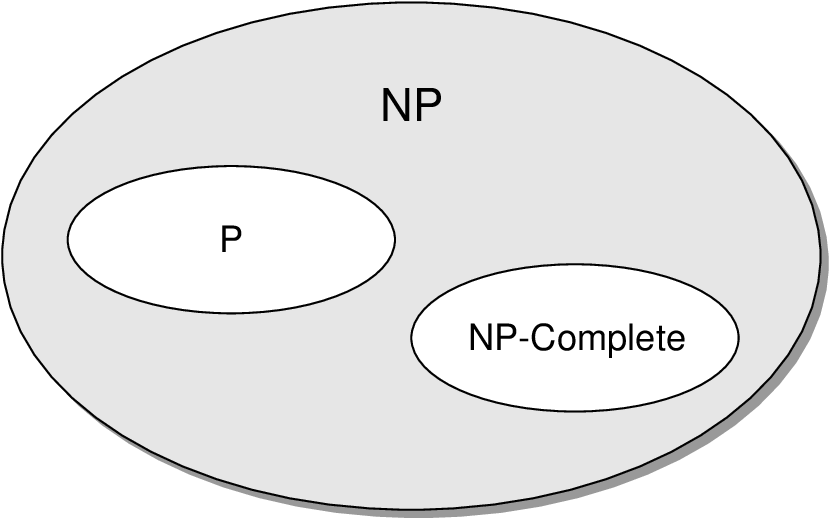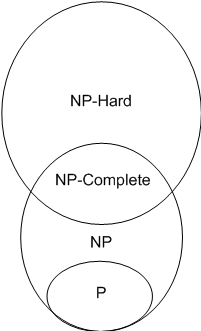×

Search anything:

# NP Complete Complexity

#### Algorithms np completeGet this book -> Problems on Array: For Interviews and Competitive Programming

In this article, we have explored the idea of NP Complete Complexity intuitively along with problems like Clique problem, Travelling Salesman Problem and more. In simple terms, a problem is NP Complete if a non-deterministic algorithm that be designed for the problem to solve it in polynomial time O(N^K) and it is the closest thing in NP to P.

All problems cannot be solved in polynomial time complexity (like O(N^2)). For example, Alan Turing's famous halting problem cannot be solved by any computer no matter how much time is provided. There are also problems that can be solved but not in polynomial time or O(n^k) time for any constant k.

Then there are problems which can verify whether a particular input is a solution of the problem or not in polynomial time O(N^K) but the determining the solution itself takes exponential time.

All the problems in computer science are categorized into multiple sets like P, NP, NP hard, NP complete. Each set has its own characteristics and there exist some interesting relations between all these sets.

Polynomial time refers to time complexities such as O(n),O(n^2),O(n^100) i.e O(n^k) for any constant k etc.

Exponential time complexity, on the other hand, refers to time complexities such as O(2^n) etc. It can be observed that polynomial time complexity is preferred over exponential time complexity.

Many problems with real life applications have exponential time complexity. These problems can take a lot of computational time. Exponential time complexity is not preferable as large inputs can take a lot of time to be solved for. Most exponential t.c problems can be widely used in real life situations and hence a polynomial solution for these problems is keenly awaited.

We try to reduce the time complexity of these problems to polynomial time. This is the esscence of NP Complexity.

NP problems are those problems in computer science which neither have a polynomial time solution nor are they confirmed to not have a polynomial time complexity.

However NP problems can be verified in polynomial time i.e given a solution we can check if the solution really satisfies the problem or not. Finding this solution however, would require exponential time.

Let us now understand a few terms which will be encountered multiple times in this article and are important for understanding NP Complexity as a concept.

Decision problems

A problem whose answer/output can only be yes or no. A decision problem is a yes-or-no question on an infinite set of inputs. It is traditional to define the decision problem as the set of possible inputs together with the set of inputs for which the answer is yes.

Deterministic and Non Deterministic Algorithms
Non deterministic algorithms can produce different outputs in each run for the same input.Its implementation cannot be written in code. For example, for searching algorithms, the best known algorithm is is of tc O(n) but suppose an algorithm is developed on paper which says that searching can be done in O(1) time. This algorithm may not be easy to write in code and hence it is assumed to be a non deterministic.
Deterministic algorithm is an algorithm which gives the same output each time for a particular input. It can be run efficiently on a machine. Most importantly, it can be implemented in code.

## Types of Problems in Computer Science

1. P: Set of decision problems which have deterministic algorithms and have polynomial time complexities.
2. NP: Set of decision problems which have non-deterministic algorithms and take polynomial time. NP refers to Non-deterministic Polynomial time.
3. NP Hard: NP-hard problems are essentially those that are at least as hard as the hardest NP problem, but don’t need to be verifiable in polynomial time.
4. NP Complete: An NP problem is considered NP Complete if a non deterministic algorithm can be written for it. A problem is NP complete has the property that it can be solved in polynomial time if and only if all other NP complete problems can be solved in polynomial time.

### NP Complete

This section throws light on NP complete problems and their characteristics. We have discussed a couple of NP complete problems below. Check them out to get a better understanding of the concept.
Definition of NP-Completeness
A language L is NP-complete if it satisfies two conditions

1. L is in NP
2. Every A in NP is polynomial time reducible to L.

If a language satisfies the second property, but not necessarily the first one, the language B is known as NP-Hard. Informally, a search problem B is NP-Hard if there exists some NP-Complete problem A that Turing reduces to B.

Basically, a problem is called NP complete when it has been reduced to a NP hard problem and has a non deterministic algorithm written for it. NP complete problems can be considered closest to being P problems. We just need to determine the non deterministic algorithm to make the problem a polynomial time problem.

Some unique points about NP Complete Problems:

• NP Complete must be both NP and NP-hard.
• It is exclusively Decision problem .

Now,let us understand another important concept which is Reduction. This is used widely in proving NP complexity of a new problem.

#### Reduction

Suppose that we have have a procedure that transforms any instance a of A into some instance b of B with the characteristics:

1. The transformation takes polynomial time
2. The answers are the same. That is, the answer for a is 'yes'if and only if the answer for b is also 'yes'.
Reduction is transitive.

### Relation between P and NPP is contained in NP.
NP complete belongs to NP
NP complete and P are exclusiveHow to relate them together?

1. Using a base problem which is CNF satisfiability or circuit satisfiability.
CNF propositional clauses formula
Xi = {x1,x2,x3}
CNF problem is :
{x1 U x2' U x3} {x1' U x2 U x3'}
We need to find the values for which CNF is satisfied
We make a state space tree for the same taking boolean values for x1,x2,x3 qnd detreming which values of x1,x2,x3 lead to True result.

Now,Let satisfiability be NP hard and all others be Hard.
The CNF problem is converted into the problem at hand which in turn makes this problem NP hard.
2) Satisfiabity has a non deterministic algorithm and is also NP hard. It's ND algo is known and hence it is NP Complete.

## Examples

### The Clique Problem

A clique in an undirected graph G = (V,E) is a subset V' in V of vertices,each pair of which is connected by an edge in E. In other words, a clique is a complete subgraph of G. The size of a clique is the number of verices it contains. The clique clique problem is the optimization of finding a clique of maximum size in a graph. As a decision problem, we ask simply whether a clique of a given size k exists in the graph. Formally,
CLIQUE = {(G,K) : G is a graph with a clique of size k}
SAT == CDP?
Let x1,x2,x3 be 3 varian=bles in SAT
Then, let F = (x1 U x2) ^ (x1' U x2') ^ (x1 U x3)
Rules for clique :

1. We cannot connect nodes that belong to the same clause
2. We cannot connect a node with its negation
For CDP, each variable and its negation is a vertex in the graph G and the above mentioned rules apply to that graph.
If you consider the rules, make a clique

F = (0 U 1) ^ (1 ^ 0) ^ (0 U 1)
Pick clique from the graph, select the vertices and use the values to satisfy SAT,it will always come out to be true.
Hence, This exercise morphs CDP to SAT

### Hamiltonian Cycle Problem(NP complete problem)

A hamiltonian cycle of an undircted graph G = (V,E) is a simple cycle that contains each vertex in V. A graph that contains a hamiltonian cycle is said to be hamiltonian otherwise it is non hamiltonian
HC = {(G) : G is a hamiltonian cycle}

### Traveling Salesman Problem(NP complete problem)

In the travelling salesman problem,a salesman must visit n cities. Modelling the problem as a complete graph with n vertices, we ca say that the salesman wisj=hes to make a tour, or a hamiltonian cycle, visisting each city ecxactly once and finishing at the city he starts from. There is an integer cost c(i,j) to travel from city i to city j, and the salesman wishes to make the tour whose total cost is minimumwhere the total cost is the sum of edges along the edges of the tour.
Formally,
TSP = {(G,c,k): G = (V,E) is a complete graph
c is a function from VxV -> Z
k belongs to Z and
G has a travelling salesman tour with cost at most k}

To prove TSP is NP hard,we reduce it to hamiltonian cycle problem.Let G = (V,E) be an instance of HAM_CYCLE. We construct an instance of TSP as follows. We form the complete graph G'= (V,E') where E'= {(i,j) : i,j belongs to V and i != j},we define the cost function by,
c(i,j) = 0 if (i,j) belongs to E
1 if (i,j) does not belong to E
We now show that graph G has a hamiltonian cycle if and only if graph G' has a tour of cost at most 0. Suppose that graph G has a hamiltonian cycle h. Each edge in h belongs to E and thus has cost 0 in G'. Thus, h is a tour in G' with cost 0.Conversely, suppose that graph G' has a tour h'of cost at most 0. Since the costs of edges in E'are 0 and 1, the cosy=t of tour h'is exactly 0 and each edge on the tour must have cost 0. Therefore, h' contains only edges in E. We conclude that h'is a hamiltonian cycle in graph G.

## P=NP?

Whether P = NP, is an unsolved problem in computer science. We are sure that P is a subset of NP but it is still unknown whether it is proper or improper subset.
If P is considered a proper subset of NP i.e Some problems in NP do not belong to P and hence are unsolvable in polynomial time.
On the other hand if we consider that P is an improper subset of NP i.e all problems of NP lie in P also,then that implies that all problems are solvable but we do not have the solutions and they need to be rediscovered.
While most researchers believe that P != NP there isn't proper proof to consider it true.

### Interesting Facts

• If any one of the NP complete problems can be solved in polynomial time, then all of them can be solved.
• If an NP Hard Problem can be solved in polynomial time,then all NP complete problems can be solved in polynomial time.